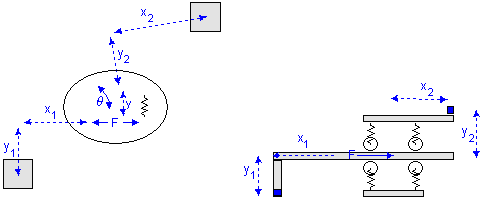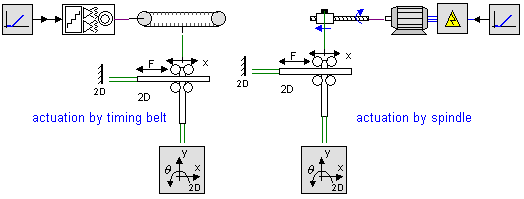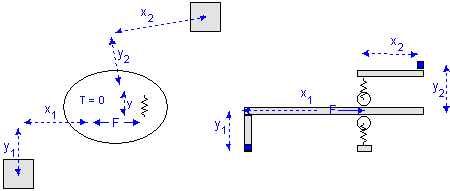﻿ 20-sim webhelp > Library > Iconic Diagrams > Mechanical > Translation > 2DSmallRotation > twodlinearactuator

# twodlinearactuator

Navigation:  Library > Iconic Diagrams > Mechanical > Translation > 2DSmallRotation >

# twodlinearactuator## Library

Iconic Diagrams\Mechanical\Translation\2DSmallAngles

X

Y

XTheta

YTheta

## Use

Domains: Continuous. Size: 1-D / 2-D. Kind: Iconic Diagrams (1D Translation, 2D Planar).

## Description - X

The TwoDLinearActuator-X model represents a flexible connection between two bodies in y- and q-direction. The TwoDLinearActuator-X model is based on the TwoDLinearSlide-X model. Instead of using a zero force in the x-direction, there is a coupling with a 1D translation port for the actuator force.

Consider a carriage that is clamped with four wheels to a slide. In the x-direction the carriage can be moved by a certain force. The limited stiffness of the wheels is represented by springs. If there is a force with equal sign on both wheels, the carriage will slightly move in the y-direction. If there is a force with opposite sign on both wheels, the carriage will only rotate. This can be represented by two independent springs: one in the y-direction and q-direction.Linear slide: x = free, y = stiff, q = stiff.

For a realistic representation of vibration behavior, every spring has a damper in parallel. If you want to turn off the effects of this damper, choose the damping parameters equal to zero. If you want to damp out unwanted vibrations, choose the damping parameters equal to 0.1% to 10% of the spring parameters.

With the 1D port connection every possible form of actuation can be created using the models from the standard library. Two examples are shown below.## Interface - X

 Ports Description P p Port with three degrees of freedom (x, y, q). 1D translation port Causality fixed force out P Parameters x1 distance in x-direction from center of stiffness to port 1 [m] y1 distance in y-direction from center of stiffness to port 1 [m] x2 distance in x-direction from center of stiffness to port 2 [m] y2 distance in y-direction from center of stiffness to port 2 [m] ky stiffness in y-direction [N/m] kth rotational stiffness [N.m/rad] dy damping in y-direction [N.s/m] dth rotational damping [N.m.s/rad] Initial Values y_initial The initial extension of the spring [m]. theta_initial The initial rotation of the spring [rad].

## Description - Y

The TwoDLinearActuator-Y model is equal to the TwoDLinearActuator-X model except that here the force acts in the y-direction.

## Interface - Y

 Ports Description P p Port with three degrees of freedom (x, y, q). 1D translation port Causality fixed force out P Parameters x1 distance in x-direction from center of stiffness to port 1 [m] y1 distance in y-direction from center of stiffness to port 1 [m] x2 distance in x-direction from center of stiffness to port 2 [m] y2 distance in y-direction from center of stiffness to port 2 [m] kx stiffness in x-direction [N/m] kth rotational stiffness [N.m/rad] dx damping in x-direction [N.s/m] dth rotational damping [N.m.s/rad] Initial Values x_initial The initial extension of the spring [m]. theta_initial The initial rotation of the spring [rad].

## Description - XTheta

The TwoDLinearActuator-XTheta model represents a flexible connection between two bodies in y-direction. The TwoDLinearActuator-XTheta model is based on the TwoDLinearSlide-XTheta model. Instead of using a zero force in the x-direction, there is a coupling with a 1D translation port for the actuator force.

Consider a carriage that is clamped with two wheels to a slide. In the x-direction the carriage can be moved by a certain force. The carriage is free to rotate, which is equal to a zero torque. The limited stiffness of the wheels is represented by springs. If there is a force acting on both wheels, the carriage will slightly move in the y-direction. If there is a force acting on the wheels, the carriage will slightly move in the y-direction.Linear slide: x = free, y = stiff, q = stiff.

For a realistic representation of vibration behavior, the spring has a damper in parallel. If you want to turn off the effects of this damper, choose the damping parameters equal to zero. If you want to damp out unwanted vibrations, choose the damping parameter equal to 0.1% to 10% of the spring parameter.

With the 1D port connection every possible form of actuation can be created using the models from the standard library. For example see the TwoDLinearActuator-X model.

## Interface - XTheta

 Ports Description P p Port with three degrees of freedom (x, y, q). 1D translation port Causality fixed force out P Parameters x1 distance in x-direction from center of stiffness to port 1 [m] y1 distance in y-direction from center of stiffness to port 1 [m] x2 distance in x-direction from center of stiffness to port 2 [m] y2 distance in y-direction from center of stiffness to port 2 [m] ky stiffness in y-direction [N/m] dy damping in y-direction [N.s/m] Initial Values y_initial The initial extension of the spring [m].

## Description - YTheta

The TwoDLinearActuator-YTheta model is equal to the TwoDLinearActuator-XTheta model except that here the force acts in the y-direction.

## Interface - YTheta

 Ports Description P p Port with three degrees of freedom (x, y, q). 1D translation port Causality fixed force out P Parameters x1 distance in x-direction from center of stiffness to port 1 [m] y1 distance in y-direction from center of stiffness to port 1 [m] x2 distance in x-direction from center of stiffness to port 2 [m] y2 distance in y-direction from center of stiffness to port 2 [m] kx stiffness in x-direction [N/m] kth rotational stiffness [N.m/rad] dx damping in x-direction [N.s/m] dth rotational damping [N.m.s/rad] Initial Values x_initial The initial extension of the spring [m]. theta_initial The initial rotation of the spring [rad].